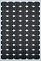• Introduction
• What is in this manual
• What is Caspoc
• User interface
• Introduction
• Starting
• Simulation
• Editing
• Viewing and printing
• Getting Started
• Basic editing
• Simulation in the time domain
• Basic User Interface Topics
• Editing
• Simulation
• Viewing
• Library
• Reports
• Project management
• Circuit and Block Diagram Components
• Introduction
• Cscript and user defined functions
• Component parameters
• Modeling Topics
• Introduction
• Power Electronics
• Semiconductors
• Electrical Machines
• Electrical drives
• Power Systems
• Mechanical Systems
• Thermal Systems
• Magnetic Circuits
• Green Energy
• Coupling to FEM
• Experimenter
• Analog hardware description language
• Embedded C code Export
• Coupling to Spice
• Small Signal Analysis
• Matlab coupling
• Tips and tricks
• Appendices

## Solar Module parameters

Modeling a Solar Cell based on the Voltage/Current characteristic. This model is more detailed than the solar cell model and can be used for maximum power point tracking. Although the model requires is more complicated than the solar cell model, it gives more accurate results.The parameters for the solar module are given in the table below. The model is based on the voltage-current characterisitic and also includes the parasitic circuitry surrounding the solar module.

Compared to the solar cell model, the solar module model can be defined for any nominal operating temperature (Tn) and sunlight density (Enom)

The parameter Factor can be used to enhance the simulation, in almost any case you can leave it to the default value of 10

The parasitic circuitry is defined by the parameters Cparallel, Rparallel, Rseries, Lseries

Specify the open circuit voltage (Uoc), short circuit current (Isc), maximum power point voltage (Umpp) and maximum power point current (Impp) for the nominal Temperature (Tn) and nominal sunlight density (Enom)

The open-circuit voltage temperature coefficient αU specfies the temperature dependence. for the voltage of the characteristic. The short-circuit current temperature coefficient αI specfies the temperature dependence. for the current of the characteristic.

default values are given below for a 150Watt solar module

• Nominal Voltage 24 [V]
• Maximum Power Point MPP 150[W]
• Rated current IMPP 4.4 [A]
• Rated voltage VMPP 34.0 [V]
• Short circuit current ISC 4.8 [A]
• Open circuit voltage VOC 43.4 [V]

 Parameter Description Default value Cparallel Parallel Cell Capacitance 1nF Enom[W/m2] Nominal energy density 1000 Factor[10 .. 100] Simulation factor, set ot 10, or higher 10 Impp[A] Current at maximum power point 4.4 Isc[A] Current at short circuit 4.8 Lseries[H] Series wire inductance of the connection leads. 1n Rparallel[Ohm] Parallel leakage resistance 100 Rseries[Ohm] Series wire resistance of the connection leads. 20m Tn[Celcius Nominal operation temperature 25 Umpp[V] Voltage at maximum power point 34 Uoc[V] Voltage at open circuit 43.4 αI[A/Celcius] Temperature coefficient for the current 2.06m αU[V/Celcius] Temperature coefficient for the voltage -0.152

The inputs on the left side are the sunlight density and the temperature. The terminals on the top and bottom are the electrical terminals. The connection on the right side is for monitoring the internal variables of the solar module and is not used.